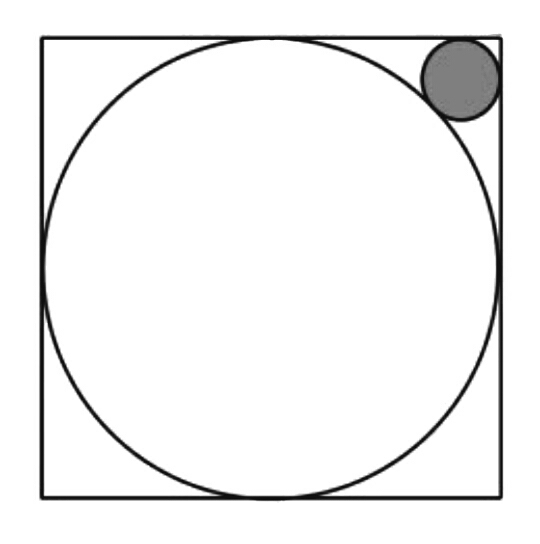# Beauty of Geometry - 1 (Easier version)

Geometry Level 3There is a circle inscribed in a square. Inside the square, where the inscribed circle isn't present, we inscribe another small circle (shaded) touching both of the square and the bigger circle.

If the radius of the bigger circle is 15 cm, then what will be the radius of the smaller circle (in cm)? Round your answer to 3 decimal digit.

You can find the original problem here.

×